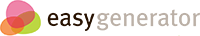# Physics

## Untitled section

### 1. Which mode(s) of ultrasonography provides a direct information about the amplitude of echoes:

• B – mode only
• A-mode only
• A and B modes
• A and M modes
• M – mode only

### 2. After the light absorption by a photoreceptor the membrane potential is:

• A) Hyperpolarized due to closure of cation selective channels activated by cyclic GMP
• A) Depolarized due to opening of voltage-gated sodium channels
• A) Hyperpolarized due to opening of voltage-gated calcium channels
• A) Depolarized due to opening of cation selective channels activated by cyclic AMP
• Hyperpolarized due to closure of cation selective channels activated by cyclic AMP

### The force of electrostatic interaction between two electric charges is:

• proportional to the product of the two electrical charges
• Proportional to the distance between electrical charges
• inversely proportional to the distance between electrical charges
• Proportional to the square of the distance between electrical charges
• Inversely proportional to the sixth power of the distance

### 4) The energy ofd is:

• Inversely proportional to the sixth power of the distance
• Proportional to the square of the distance between electrical charges
• Proportional to the distance between electrical charges
• Inversely proportional to the distance between electrical charges
• Inversely proportional to the square of the distance between electrical charges

### 5. Van der Waals attractive interactions are due to

• dipole interactions
• hydrogen bonds
• short distance strong (rigid) repulsion
• covalent reactions
• energy supplied by external force when decreasing the system entropy

### 6) Radiation-induced ionization of gas occurs in:

• Geiger counter
• scintillation counter
• photographic film
• galvanometer
• semiconductor counter

### 7) The sample contains 2000 atoms of radioactive isotope whose half-life is 50 years. Radioactive decays will reduce the initial number of radioactive atoms to 125 after:

• A. 200 years
• A. 100 years
• A. 187,5 years
• A. 250 years
• A. 375 years

### 8) During MRI scan a patient is placed in the constant magnetic field of a scanner and the protons in patient’s body absorb pulses of electromagnetic radiation whose frequency is typical for:

• A. radio waves
• A. X-ray
• A. infrared
• A. ultraviolet
• A. microwaves

### 9) In ultrasonography reflections of ultrasound waves (echos) may occur at the boundary between two tissues:

• A. if both tissues have dissimilar acoustic resistances
• A. if both tissues have the same acoustic resistances
• A. if both tissues have the same densities
• A. if the boundary is very close to a transducer
• A. if the boundary lays inside near field of a transducer

• A. 8 s
• A. 2 s
• A. 4 s
• A. 1.5 s
• A. 3 s

### 11) The NMR phenomenon is related to:

• absorption of quanta of electromagnetic radiation correlated with change in the spin moment orientation
• emission of ultrafiolet radiation
• emission of gamma rays from atomic nuclei
• absorption of infrared radiation
• absorption of energy carried by ultrasounds

### 12) The mean kinetic energy of particles in an ideal gas is:

• proportional to the temperature
• inversely proportional to the temperature
• inversely proportional to the pressure
• proportional to the pressure
• proportional to the volume